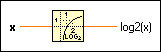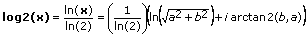# Logarithm Base 2 Function

## LabVIEW 2018 Help

Edition Date: March 2018
Part Number: 371361R-01
View Product InfoLabVIEW 2016 HelpLabVIEW 2017 HelpLabVIEW 2018 HelpLabVIEW 2019 HelpLabVIEW 2020 Help

Owning Palette: Exponential Functions

Requires: Base Development System

Computes the base 2 logarithm of x.

If x is 0, log2(x) is negative infinity. If x is not complex and is less than 0, log2(x) is NaN. The connector pane displays the default data types for this polymorphic function.

Examplex can be a scalar number, array or cluster of numbers, array of clusters of numbers, and so on.log2(x) is of the same numeric representation as x. When x is of the form x = a + bi, that is, when x is complex, the following equation defines log2(x):## Example

Refer to the Exponential VI in the labview\examples\Mathematics\Elementary & Special Functions\Exponential Functions directory for an example of using the Logarithm Base 2 function.